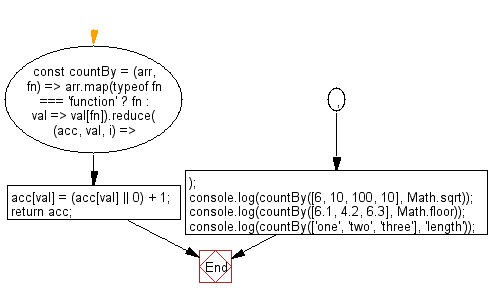# JavaScript: Group the elements of an array based on the given function and returns the count of elements in each group

## JavaScript fundamental (ES6 Syntax): Exercise-69 with Solution

Write a JavaScript program to group the elements of an array based on the given function and returns the count of elements in each group.

• Use Array.prototype.map() to map the values of an array to a function or property name.
• Use Array.prototype.reduce() to create an object, where the keys are produced from the mapped results.

Sample Solution:

JavaScript Code:

``````//#Source https://bit.ly/2neWfJ2
const countBy = (arr, fn) =>
arr.map(typeof fn === 'function' ? fn : val => val[fn]).reduce((acc, val, i) => {
acc[val] = (acc[val] || 0) + 1;
return acc;
}, {});
console.log(countBy([6, 10, 100, 10], Math.sqrt));
console.log(countBy([6.1, 4.2, 6.3], Math.floor));
console.log(countBy(['one', 'two', 'three'], 'length'));
```
```

Sample Output:

```{"10":1,"2.449489742783178":1,"3.1622776601683795":2}
{"4":1,"6":2}
{"3":2,"5":1}
```

Flowchart:Live Demo:

See the Pen javascript-basic-exercise-69-1 by w3resource (@w3resource) on CodePen.

Improve this sample solution and post your code through Disqus

What is the difficulty level of this exercise?

Test your Programming skills with w3resource's quiz.

﻿

## JavaScript: Tips of the Day

Creates an object from the given key-value pairs

Example:

```const tips_objectFromPairs = arr => arr.reduce((a, [key, val]) => ((a[key] = val), a), {});
console.log(tips_objectFromPairs([['x', 2], ['y', 4]]));
```

Output:

```[object Object] {
x: 2,
y: 4
}
```